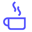a concise shot of dev knowledge
Become a Contributor
Concise shots of dev knowledge

RELATED TAGS

algorithms
dijkstra
graph

# What is Dijkstra's algorithm?Edpresso Team

Dijkstra’s Algorithm allows you to calculate the shortest path between one node of your choosing and every other node in a graph.## Algorithm Execution

Here’s how the algorithm is implemented:

1. Mark all nodes as unvisited.

2. Mark the selected initial node with a current distance of 0 and the rest with infinity.

3. Set the initial node as current node.

4. For the current node, consider all of its unvisited neighbors and calculate their distances by adding the current distance of current node to the weight of the edge connecting neighbor node and current node.

5. Compare the newly calculated distance to the current distance assigned to the neighboring node and set is as the new current distance of neighboring node.

6. When done considering all of the unvisited neighbors of the current node, mark the current node as visited.

7. If the destination node has been marked visited then stop. The algorithm has finished.

8. Otherwise, select the unvisited node that is marked with the smallest distance, set it as the new current node, and go back to step 4.

## Demo

Let’s take a look at an illustration implementing Dijkstra’s to understand the algorithm better!

1 of 31

## Uses of Dijkstra’s Algorithm

Dijkstra’s Algorithm has several real-world use cases, including the following:

• Map applications.
• Finding locations of a map referring to vertices of a graph.
• IP routing to find Open Shortest Path First.
• Telephone networks.

RELATED TAGS

algorithms
dijkstra
graph
RELATED COURSES

View all Courses

Keep Exploring

Learn in-demand tech skills in half the time